# 21.11: Pericyclic Reactions

$$\newcommand{\vecs}{\overset { \rightharpoonup} {\mathbf{#1}} }$$ $$\newcommand{\vecd}{\overset{-\!-\!\rightharpoonup}{\vphantom{a}\smash {#1}}}$$$$\newcommand{\id}{\mathrm{id}}$$ $$\newcommand{\Span}{\mathrm{span}}$$ $$\newcommand{\kernel}{\mathrm{null}\,}$$ $$\newcommand{\range}{\mathrm{range}\,}$$ $$\newcommand{\RealPart}{\mathrm{Re}}$$ $$\newcommand{\ImaginaryPart}{\mathrm{Im}}$$ $$\newcommand{\Argument}{\mathrm{Arg}}$$ $$\newcommand{\norm}{\| #1 \|}$$ $$\newcommand{\inner}{\langle #1, #2 \rangle}$$ $$\newcommand{\Span}{\mathrm{span}}$$ $$\newcommand{\id}{\mathrm{id}}$$ $$\newcommand{\Span}{\mathrm{span}}$$ $$\newcommand{\kernel}{\mathrm{null}\,}$$ $$\newcommand{\range}{\mathrm{range}\,}$$ $$\newcommand{\RealPart}{\mathrm{Re}}$$ $$\newcommand{\ImaginaryPart}{\mathrm{Im}}$$ $$\newcommand{\Argument}{\mathrm{Arg}}$$ $$\newcommand{\norm}{\| #1 \|}$$ $$\newcommand{\inner}{\langle #1, #2 \rangle}$$ $$\newcommand{\Span}{\mathrm{span}}$$

## Why are [4 + 2] and [2 + 2] Cycloadditions Different?

There are numerous reactions in organic chemistry that proceed through cyclic transition states. They may be classified generally as pericyclic reactions. An important and familiar example is the Diels-Alder reaction, in which a conjugated diene cycloadds to an alkene or alkyne: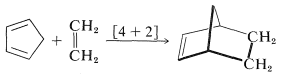This reaction has been described previously (Section 13-3A) and is an example of a [4 + 2] cycloaddition. Such reactions occur thermally (by simply heating the reactants) and appear to be entirely concerted. By this we mean that the reactants are converted to products in one step, without involving the formation of reaction intermediates. The principal evidence for the concertedness of [4 + 2] cycloadditions is the fact that they are highly stereospecific and involve suprafacial addition of both components. The configuration of substituents in the diene and the dienophile is retained in the adduct:In contrast to the [4 + 2] cycloaddition, thermal [2 + 2] cycloadditions seldom are observed, and when they are observed, they are not stereospecific and evidently are stepwise reactions (see Section 21-11):Why are the [4 + 2] and [2 + 2] cycloadditions different? Simple molecular orbital theory provides an elegant explanation of this difference based on the $$4n + 2$$ rule described in Section 21-9. To understand this, we need to look in more detail at how the $$p$$ orbitals of the double bonds interact in concerted addition mechanisms by suprafacial overlap, as in $$36$$ and $$37$$:Figure 21-5) and, for $$37$$, a set of four cyclobutadienelike molecular orbitals ($$29$$). The $$4n + 2$$ rule applies to such systems, and a transition state such as $$36$$, which has six electrons, therefore can be very much more favorable than one such as $$37$$, which has four electrons. From this we can conclude that concerted cycloaddition reactions involving $$\left( 4n + 2 \right)$$ $$\pi$$ electrons generally should be more favorable than those involving $$4n$$ $$\pi$$ electrons. Indeed, transition states such as $$37$$ generally are less favorable than transition states for formation of biradicals or ions (Section 21-11).

## How Mr. Möbius Beat the $$4n + 2$$ Rule

There is a way around the $$4n + 2$$ rule that is not very important for substances analogous to benzene, but is quite important for cycloaddition reactions. Let us see how this works for a cyclic conjugated polyene.

From the molecular-orbital diagrams of Figures 21-5, 21-7, 21-9, and 21-14, you will see that the lowest-energy $$\pi$$ molecular orbital has no nodes (changes of phase). A model of such an orbital, which usually is called a Hückel orbital, can be constructed by joining the ends of a ribbon or strip of parallel $$p$$ orbitals, as represented on the left side of Figure 21-15. However, one could join the orbitals by making one twist in the strip, which then would give a lowest-energy orbital with one node, as on the right side of Figure 21-15. A strip with one such twist is called a Möbius strip$$^8$$ and has the topological property of having only one side.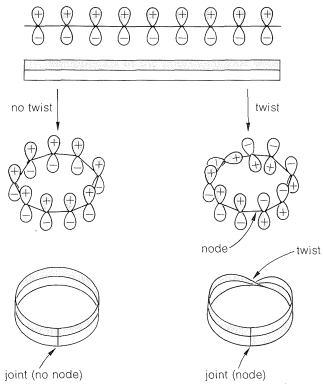Figure 21-15: Normal (Hückel) and Möbius rings of $$\pi$$ orbitals. To clarify the difference between the two rings, visualize a strip of black-red typewriter ribbon, the black representing the $$+$$ phase, and the red the $$-$$ orbital phase. Now join the ends together without, or with, one twist in the strip. At the joint there then will be no node (left) or one node (right).

If we now calculate the orbital energies for the Möbius orbitals, as was down for the normal Hückel $$\pi$$ orbitals in Figure 21-13, we get the results shown in Figure 21-16. From this, we see that the $$4n$$ situation now is favored and $$4n + 2$$ is unfavorable. Whereas the energies of the $$\pi$$ molecular orbitals in the Hückel arrangement can be obtained by inscribing a polygon in a circle with a corner down (Section 21-9A), in the Möbius arrangement the orbital energies are obtained from the polygon inscribed with a side down.Figure 21-16: Calculated $$\pi$$-molecular orbital energies for $$N$$ overlapping $$p$$ orbitals in Möbius cyclic $$\pi$$ systems.

If you compare the orbital energies of the Hückel and Möbius cyclic $$\pi$$ systems (Figures 21-13 and 21-16), you will see that the Hückel systems have only one lowest-energy MO, whereas the Möbius systems have two. Hückel systems have an odd number of bonding orbitals (which, when full, accommodate 2, 6, 10, 14, or $$4n + 2$$ electrons) and the Möbius systems have an even number of bonding orbitals (which, when full, accommodate 4, 8, 12, or $$4n$$ electrons). The Hückel molecular orbitals have zero or an even number of nodes (see, for example, the benzene MOs, Figure 21-5); the Möbius molecular orbitals are not shown, but they have one or an odd number of nodes.

The relevance of all this may seem tenuous, especially because no example of a simple cyclic polyene with a Möbius $$\pi$$ system is known. However, the Möbius arrangement is relevant to cycloaddition because we can conceive of alkenes, alkadienes, and so on approaching each other to produce Möbius transition states when $$4n$$ electrons are involved.

For example, consider two molecules of ethene, which we showed previously would violate the $$4n + 2$$ rule by undergoing cycloaddition through a transition state represented by $$37$$. There is an alternative transition state, $$38$$, in which the four $$p$$ orbitals come together in the Möbius arrangement (with one node for minimum energy).

To achieve this arrangement the ethene molecules approach each other in roughly perpendicular planes so that the $$p$$ orbitals overlap suprafacially in one ethene and antarafacially in the other, as shown in $$38$$:This pathway is electronically favorable, but the steric interference between the groups attached to the double bond is likely to be severe. Such repulsions can be relieved if there are no groups sticking out sidewise at one end of the double bond, as with the central carbon of 1,2-propadiene, $$\ce{CH_2=C=CH_2}$$, and ketene, $$\ce{CH_2=C=O}$$. These substances often undergo [2 + 2] cycloadditions rather readily (Section 13-3D), and it is likely that these are concerted additions occurring by the Möbius route.

A much less strained Möbius [4 + 4] transition state can be formed from two s-cis molecules of 1,3-butadiene. When 1,3-butadiene is heated by itself, a few percent of 1,5-cyclooctadiene is formed, but it is not known for sure whether the mechanism is that shown: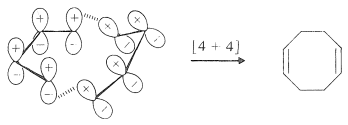The principal reaction is a Diels-Alder [4 + 2] cycloaddition, with butadiene acting both as a diene and as a dienophile:## Orbital Symmetry. The Woodward-Hoffmann Rules

Much of what we have said about the electronic factors controlling whether a cycloaddition reaction can be concerted or not originally was formulated by the American chemists R. B. Woodward and R. Hoffmann several years ago, in terms of what came to be called the orbital symmetry principles, or the Woodward-Hoffmann rules. Orbital symmetry arguments are too complicated for this book, and we shall, instead, use the $$4n + 2$$ electron rule for normal Hückel arrangements of $$\pi$$ systems and the $$4n$$ electron rule for Möbius arrangements. This is a particularly simple approach among several available to account for the phenomena to which Woodward and Hoffmann drew special attention and explained by what they call "conservation of orbital symmetry".

## Electrocyclic and Sigmatropic Rearrangements

The cycloaddition reactions that we have discussed so far in this chapter ([2 + 2], [4 + 2], etc.) have involved ring formation by bringing two unsaturated molecules together. Thus [4 + 2] addition is represented by the Diels-Alder reaction of ethene and 1,3-butadiene:We can conceive of similar cyclizations involving only single molecules, that is, intramolecular cyclization. Such reactions are called electrocyclic rearrangements. Two examples follow to show cyclization of a diene and a triene: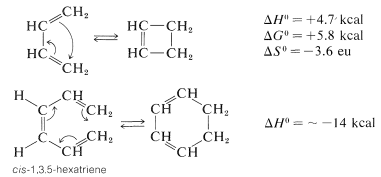Cyclization of 1,3,5-hexatriene occurs only when the central double bond has the cis configuration. The reaction is reversible at elevated temperatures because of the gain in entropy on ring opening (see Section 4-4B). The cyclobutene-1,3-butadiene interconversion proceeds much less readily, even in the thermodynamically favorable direction of ring opening. However, substituted dienes and cyclobutenes often react more rapidly.

A related group of reactions involves shifts of substituent groups from one atom to another; for example, with $$\ce{H}$$, alkyl, or aryl groups as $$\ce{R}$$:These reactions are called sigmatropic rearrangements and, in general, they are subject to the $$4n + 2$$ rule and the Möbius orbital modification of it. Potential sigmatropic rearrangements can be recognized by the fact that the single bond to the migrating group $$\left( \ce{R} \right)$$ is "conjugated" with the $$\pi$$ bonds, and the group moves from a saturated $$sp^3$$ to an $$sp^2$$ carbon at a different part of the $$\pi$$ system.

## The Stereochemistry of Electrocyclic Rearrangements

A striking feature of thermal electrocyclic reactions that proceed by concerted mechanisms is their high degree of stereospecificity. Thus when cis-3,4-dimethylcyclobutene is heated, it affords only one of the three possible cis-trans isomers of 2,4-hexadiene, namely, cis,trans-2,4-hexadiene:We can see how this can occur if, as the ring opens, the ends of the diene twist in the same direction ($$\curvearrowright \curvearrowright$$ or $$\curvearrowleft \curvearrowleft$$, conrotatory) as indicated in the equation. You will notice that with this particular case, if conrotation occurs to the left, rather than the right, the same final product results:The conrotatory movement of groups is typical of thermal ring openings of cyclobutenes and other rings involving $$4n$$ electrons.

When a cyclobutene is so constituted that conrotation cannot occur for steric reasons, then the concerted reaction cannot occur easily. Substances that otherwise might be predicted to be highly unstable often turn out to be relatively stable. An example is bicyclo[2.1.0]-2-pentene, which at first sight might seem incapable of isolation because of the possibility of immediate arrangement to 1,3-cyclopentadiene. This rearrangement does occur, but not so fast as to preclude isolation of the substance:How can we explain the fact that this substance can be isolated? The explanation is that, if the reaction has to be conrotatory, then the product will not be ordinary 1,3-cyclopentadiene, but cis,trans-1,3-cyclopentadiene - surely a very highly strained substance. (Try to make a ball-and-stick model of it!) This means that the concerted mechanism is not favorable:It is of great interest and importance that, with systems of $$4n + 2$$ electrons, the groups move in opposite directions ($$\curvearrowleft \curvearrowright$$ or $$\curvearrowright \curvearrowleft$$, disrotatory). For example,In this case, the disrotation of the groups toward one another would lead to the cis,cis,cis product. Because this product is not formed, it seems likely that rotation of the methyl groups toward each other must be sterically unfavorable:How can we account for the stereoselectivity of thermal electrocyclic reactions? Our problem is to understand why it is that concerted $$4n$$ electrocyclic rearrangements are conrotatory, whereas the corresponding $$4n + 2$$ processes are disrotatory. From what has been said previously, we can expect that the conrotatory processes are related to the Möbius molecular orbitals and the disrotatory processes are related to Hückel molecular orbitals. Let us see why this is so. Consider the electrocyclic interconversion of a 1,3-diene and a cyclobutene. In this case, the Hückel transition state (one having an even number of nodes) is formed by disrotation, but is unfavorable with four (that is, $$4n$$) electrons:In contrast, the Möbius transition state (one having an odd number of nodes) is formed by conrotation and is favorable with four $$\left( 4n \right)$$ electrons:You will notice that the ring closure of a 1,3-diene through the favorable Möbius transition state may appear to be able to form only an antibonding arrangement of the overlapping $$\sigma$$ orbitals, which would correspond to a high-energy cyclobutene. In fact, the normal cyclobutene would be formed, because on the way down from the transition state, the phases of the orbitals that will become the $$\sigma$$ bond change to give the bonding arrangement of the $$\sigma$$ orbitals expected for the ground state. The reverse occurs in ring opening so that this reaction also can go through the favorable Möbius transition state.

The same reasoning can be extended to electrocyclic reactions of 1,3,5-trienes and 1,3-cyclohexadienes, which involve $$4n + 2$$ electrons and consequently favor Hückel transition states attained by disrotation.

## Summary of Rules for Predicting Thermally Favorable Pericyclic Reactions

The three principal types of pericyclic reactions are cycloaddition, electrocyclic rearrangement, and sigmatropic rearrangement:The factors that control if and how these cyclization and rearrangement reactions occur in a concerted manner can be understood from the aromaticity or lack of aromaticity achieved in their cyclic transition states. For a concerted pericyclic reaction to be thermally favorable, the transition state must involve $$4n + 2$$ participating electrons if it is a Hückel orbital system, or $$4n$$ electrons if it is a Möbius orbital system. A Hückel transition state is one in which the cyclic array of participating orbitals has no nodes (or an even number) and a Möbius transition state has an odd number of nodes.

We summarize here a procedure to predict the feasibility and the stereochemistry of thermally concerted reactions involving cyclic transition states. The 1,2 rearrangement of carbocations will be used to illustrate the approach. This is a very important reaction of carbocations which we have discussed in other chapters. We use it here as an example to illustrate how qualitative MO theory can give insight into how and why reactions occur:The first step of the procedure is to draw the orbitals as they are expected to be involved in the transition state. There may be several possible arrangements. There are two such arrangements, $$41$$ and $$42$$, for the rearrangement of carbocations; the dotted lines show the regions of bond-making and bond-breaking (i.e., orbital overlap):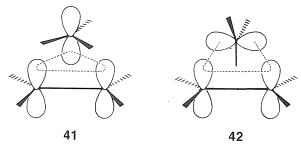The second step is to determine whether the transition states are Hückel or Möbius from the number of nodes. This is readily done by assigning signs to the lobes of the orbitals corresponding to their phases and counting the number of nodes that develop in the circle of overlapping orbitals. An odd number denotes a Möbius transition state, whereas an even number, including zero, denotes a Hückel transition state.

There are alternative ways of node-counting for transition states $$41$$ and $$42$$. Diagrams $$43abc$$ and $$44abc$$ represent molecular orbitals of different energies - those with more nodes having the higher energies (cf. Section 21-3C).$$^9$$ We show these diagrams with more than one node for the sake of completeness. It is not necessary to draw more than one such diagram to determine whether the transition state is Möbius or Hückel.Finally, we evaluate the transition states according to the $$4n$$ or $$4n + 2$$ rule. In the example here, because only two electrons occupy the molecular orbitals, the Hückel transition state ($$43a$$) is the favorable one.

A bonus coming from these formulations is that the stereochemistry of the reaction can be predicted when we have predicted which transition state is the favored one. Thus the migrating group in 1,2-carbocation rearrangements should move with retention of configuration by a Hückel transition state - and this has been verified experimentally. The alternative Möbius transition state predicts inversion of the configuration of the migrating group: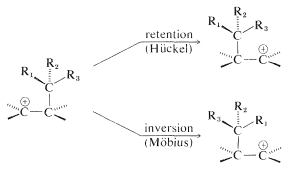You can use the procedures just outlined to determine whether any thermal reaction with a cyclic transition state is likely to be favorable. A good place to start is the Diels-Alder [4 + 2] cycloaddition, which proceeds thermally by a suprafacial (Hückel) transition state. We suggest that you apply the procedure to the Diels-Alder reaction of 1,3-butadiene and ethene, and following that, show the electrocyclic ring opening of a cyclobutene ring to be thermally favorable only by a conrotatory opening of the $$\ce{C-C}$$ bond.

## Photochemical Pericyclic Reactions

Many pericyclic reactions take place photochemically, that is, by irradiation with ultraviolet light. One example is the conversion of norbornadiene to quadricyclene, described in Section 13-3D. This reaction would have an unfavorable [2 + 2] mechanism if it were attempted by simple heating. Furthermore, the thermodynamics favor ring opening rather than ring closure. However, quadricyclene can be isolated, even if it is highly strained, because to reopen the ring thermally involves the reverse of some unfavorable [2 + 2] cycloaddition mechanism.

Photochemical activation can be used to achieve forward or reverse cycloadditions and electrocyclic reactions that are thermodynamically unfavorable or have unfavorable concerted thermal mechanisms. Thus the thermodynamically unstable disrotatory [2 + 2] product can be obtained from 1,3-cyclopentadiene by irradiation with ultraviolet light:The stereochemical results of electrocyclic and cycloaddition reactions carried out photochemically often are opposite to what is observed for corresponding thermal reactions. However, exceptions are known and the degree of stereospecificity is not always as high as in the thermal reactions. Further examples of photochemical pericyclic reactions are given in Section 28-2D.

$$^8$$Named after the mathematician A. F. Möbius.

$$^9$$The assignment of orbital phases must take appropriate account of molecular symmetry, and although this is easy for open-chain systems, it is much less straightforward for cyclic ones. You usually will be able to avoid this problem by always trying to set up the orbitals so that the transition state will have no nodes, or just one node at a point where a bond is being made or broken.